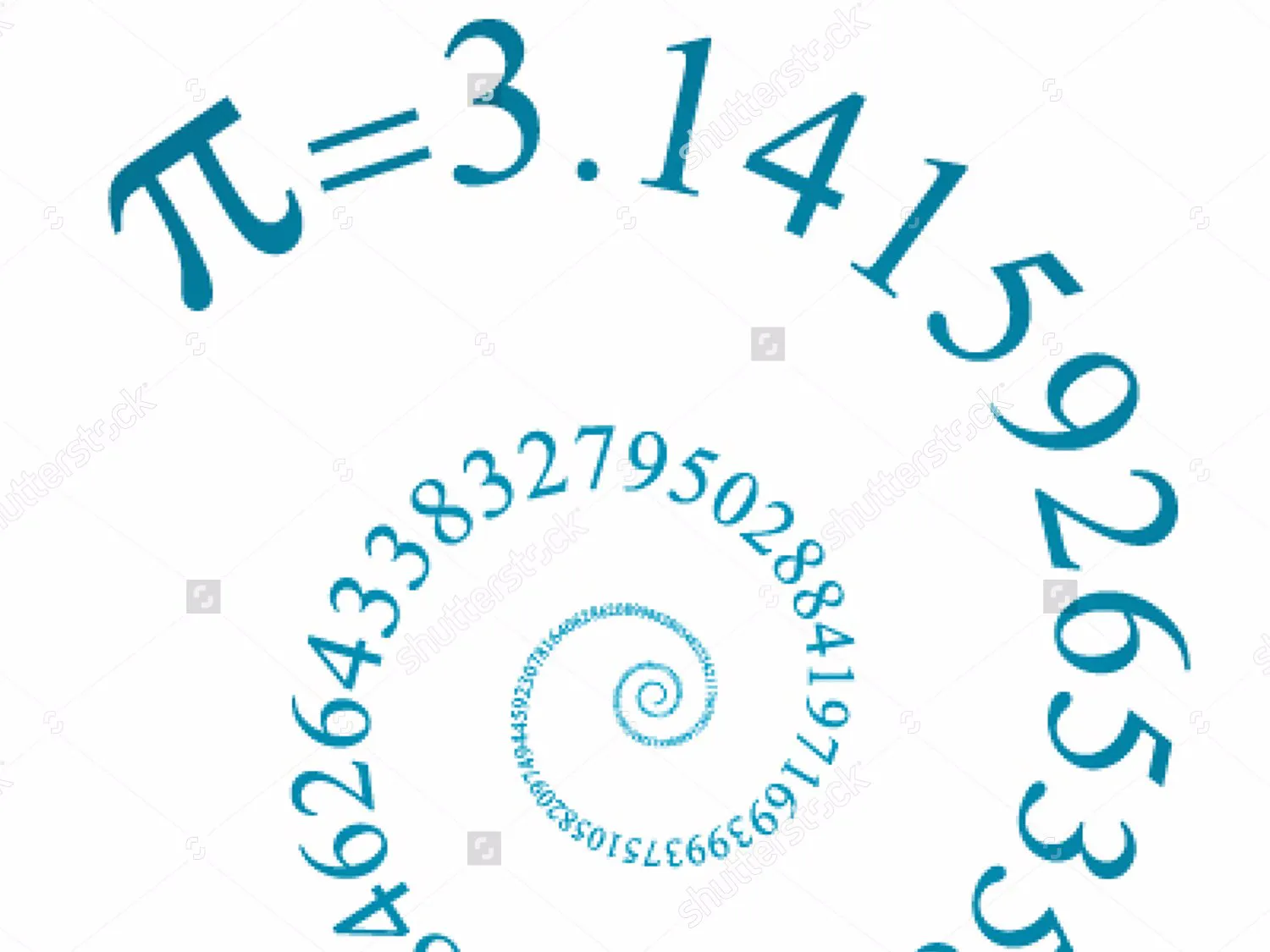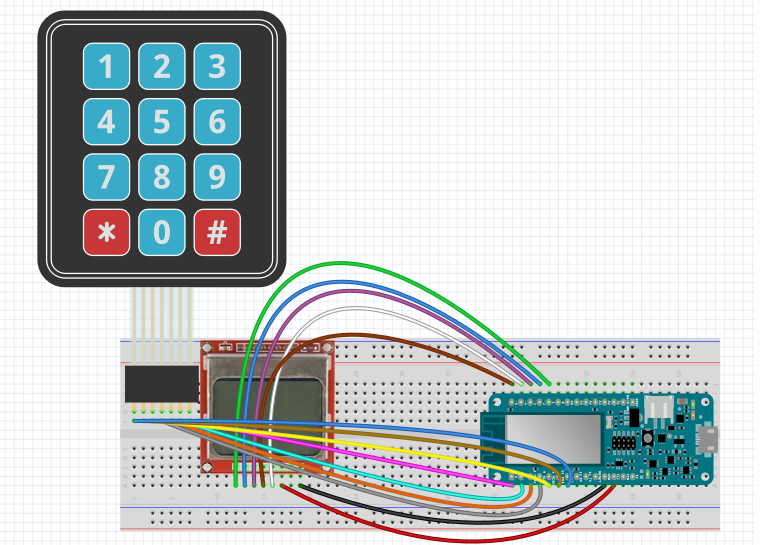# Calculating Pi

Calculating Pi number by using John Wallis series!!

• 4,624 views
• 1 comment
• 1 respect

## Components and supplies

There are many ways to calculate Pi! most common way is using one of many series that are available! a series is consist of infinite number of therms, as we use more terms of series, our Pi number will be more correct!

I want to build a device that asks user how many terms of series user want to calculate! then microcontroller will calculate Pi number as exact as we want! i want to use a series that is fined by John Wallis!

at first we have to wire our components! as is shown in schematic!

Now we have to code! for receiving numbers from key pad we write the code in loop. key pad give us chars, for converting char to integer we have to mines 48 from what we receive. and when we press *, the number of terms will go to next function!

`````` char customKey = customKeypad.getKey();
if (customKey){
if (customKey=='*'){
pi(m); //calculating pi number with m terms!!
m=0;
}
else
{
Serial.println(customKey);
m*=10;
m+=(int(customKey)-48); // convert char to int by -48!!
};
}

``````

For calculating Pi we will write a function that receive number of required terms, then calculate the Pi and show it on LCD.

``````void pi(int m){
double result=4;
for (int x=1; x<m; x++){
result*=(2*x);
result/=(2*x+1);
result*=(2*x+2);
result/=(2*x+1);
}
Serial.println(result,76);
display.clearDisplay();
display.setTextSize(1);
display.setTextColor(BLACK);
display.setCursor(0,0);
display.println(result,76); //showing pi number on lcd
display.setTextColor(WHITE, BLACK);
display.display();
}
``````

now we want to test it!!

At the first time we gave 1 to key pad, and mkr1000 calculate only one term of series! and the result will be 4!

and if we calculate 5 terms! the Pi will be 3.3023! and so on...

## Code

##### PiArduino
```int m=0;  // number of sets!

const byte ROWS = 4; //four rows
const byte COLS = 4; //four columns
//define the cymbols on the buttons of the keypads
char hexaKeys[ROWS][COLS] = {
{'1','2','3','A'},
{'4','5','6','B'},
{'7','8','9','C'},
{'*','0','#','F'}
};
byte rowPins[ROWS] = {13, 12, 11, 10}; //connect to the row pinouts of the keypad
byte colPins[COLS] = {9, 8, 7, 6}; //connect to the column pinouts of the keypad

//initialize an instance of class NewKeypad

void setup(){
Serial.begin(9600);
display.begin();
display.setContrast(50);
display.clearDisplay();
}

void loop(){

if (customKey){
if (customKey=='*'){
pi(m); //calculating pi number with m terms!!
m=0;
}
else
{
Serial.println(customKey);
m*=10;
m+=(int(customKey)-48); // convert char to int by -48!!
};
}
}

void pi(int m){
double result=4;
for (int x=1; x<m; x++){
result*=(2*x);
result/=(2*x+1);
result*=(2*x+2);
result/=(2*x+1);
}
Serial.println(result,76);
display.clearDisplay();
display.setTextSize(1);
display.setTextColor(BLACK);
display.setCursor(0,0);
display.println(result,76); //showing pi number on lcd
display.setTextColor(WHITE, BLACK);
display.display();
}
```

## Schematics

pi_kBIe4I4gpH.fzzMarch 14, 2017

#### Members who respect this project

See similar projects
you might like

#### PIR Motion Sensor: How to Use PIRs w/ Arduino & Raspberry Pi

• 101,746 views
• 47 respects

• 48,268 views
• 33 respects

• 39,497 views
• 23 respects

#### Arduino + Raspberry Pi + Ubidots Made Easy

Project tutorial by David Escobar

• 18,182 views
• 46 respects

• 16,824 views